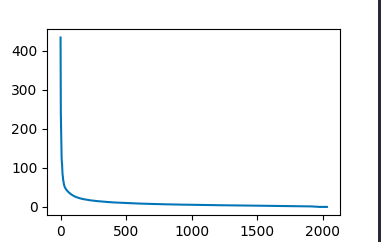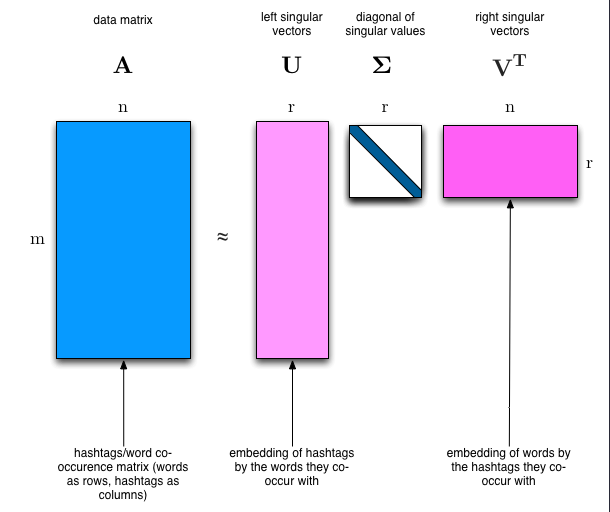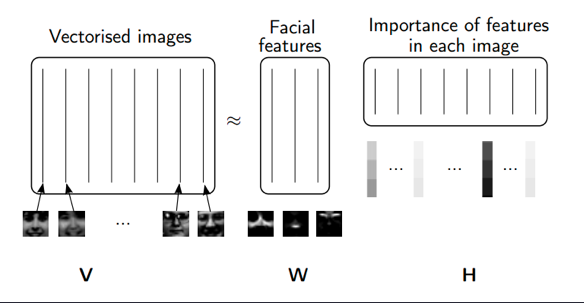# Modeling With NMF And SVD

### Why we decomposing a matrix:

Decomposing matrices into matrices that have special properties.

• SVD
• NMF

## SVD

Eg: image composition
SVD is an exact decomposition, since the matrices it creates are big enough to fully cover the original matrix(you can recover your matrix).

### Singular value:

Can give us kind of measure of importance of matrixRanking

### How to separate different topics?

We would clearly expect that the words that appear most frequently in one topic would appear less frequently in the other - otherwise that word wouldn’t make a good choice to separate out the two topics. Therefore, we expect the topics to be orthogonal(eg: columns of $U$ or rows of $V$ are orthogonal).### What the matrix means in a intuitive way?

$U$ row direction means the first column of matrix $A$, and columns means topics, they are orthogonal.
$S$ is diagonal matrix and ordered in descending order, give us a sense of importance.(topics by topics)
$V$ row direction means topics, and column direction means different words frequencies.

#how to show that the result of linalg.svd is a decomposition of the input
import numpy as np
from sklearn.datasets import fetch_20newsgroups
from sklearn import decomposition
from scipy import linalg
import matplotlib.pyplot as plt
categories = ['alt.atheism', 'talk.religion.misc', 'comp.graphics', 'sci.space']
newsgroups_train = fetch_20newsgroups(subset='train', categories=categories, remove=remove)
newsgroups_test = fetch_20newsgroups(subset='test', categories=categories, remove=remove)
num_topics, num_top_words = 6, 8
from sklearn.feature_extraction.text import CountVectorizer, TfidfVectorizer
vectorizer = CountVectorizer(stop_words='english')
vectors = vectorizer.fit_transform(newsgroups_train.data).todense() # (documents, vocab)
vectors.shape #, vectors.nnz / vectors.shape, row_means.shape
vocab = np.array(vectorizer.get_feature_names())
%time U, s, Vh = linalg.svd(vectors, full_matrices=False)
n = U @ np.diag(s) @ Vh
np.linalg.norm(n-vectors)  ## which is very small

## how to show that U and V are orthonormal
np.allclose (U @ U.T, np.eye(U.shape))
np.allclose (Vh @ Vh.T, np.eye(U.shape))

# show topics that are biggest
num_top_words=8

def show_topics(a):
top_words = lambda t: [vocab[i] for i in np.argsort(t)[:-num_top_words-1:-1]]
topic_words = ([top_words(t) for t in a])
return [' '.join(t) for t in topic_words]
show_topics(Vh[:10])


## NMF

Rather than constraining our factors to be orthogonal, another idea would to constrain them to be non-negative. NMF is a factorization of a non-negative data set $V$: $V = W H$ into non-negative matrices $W,\; H$. Often positive factors will be more easily interpretable (and this is the reason behind NMF’s popularity).

Benefits: Fast and easy to use!
Downsides: Took years of research and expertise to create

### Applications of NMF

• Face Decompositions
• Collaborative Filtering, eg movie recommendations
• Audio source separation
• Chemistry
• Bioinformatics and Gene Expression
• Topic Modeling (our problem!)### SGD

Applying SGD to NMF
Goal: Decompose $V\;(m \times n)$ into $V \approx WH$ where $W\;(m \times d)$ and $H\;(d \times n)$, $W,H > 0$, and we’ve minimized the Frobenius norm of $V-WH$.
Approach: We will pick random positive $W$ & $H$, and then use SGD to optimize.
To use SGD, we need to know the gradient of the loss function.

Welcome to share or comment on this post: